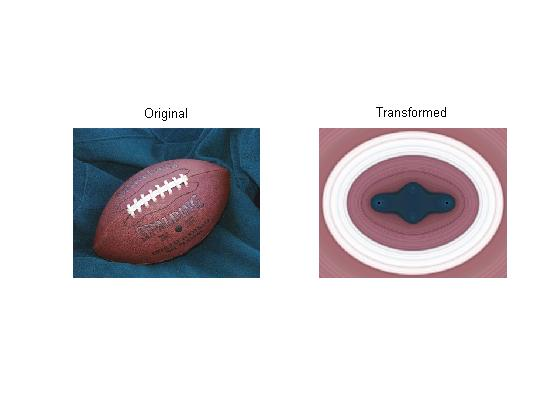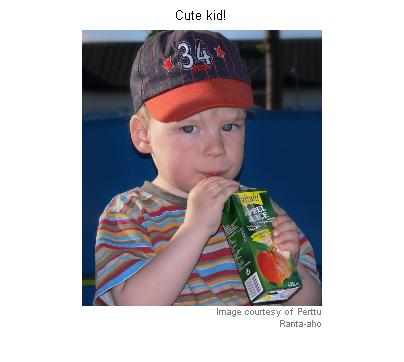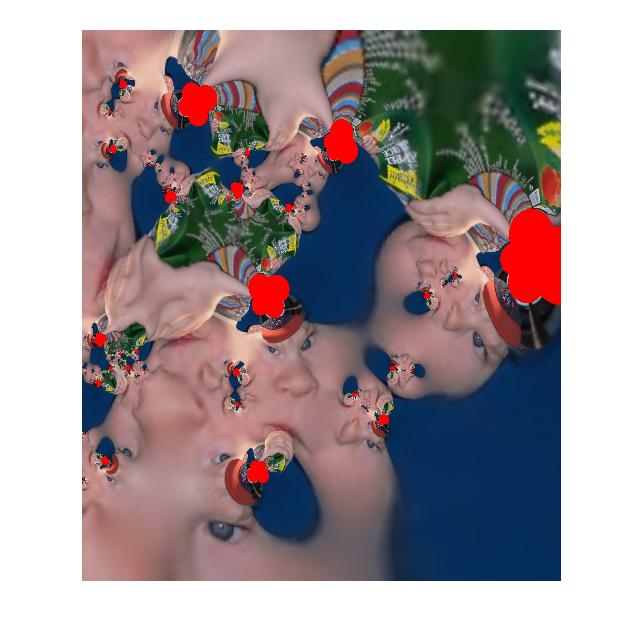## Steve on Image Processing with MATLABImage processing concepts, algorithms, and MATLAB

Thanks to Kimo Johnson and Perttu Ranta-aho for responding to my custom spatial transformation challenge.

### Kimo's submission

Kimo's custom transform turns the football image inside out!

im = imread('football.jpg');
sx = 1.47;
sy = 1.6;
c2 = 0.14;
c3 = 0.07;
p1 = 0.25;
p2 = 0.125;

f = @(x) complex(x(:,1)/sx,x(:,2)/sy);
g = @(z) c2*log(abs(z + p1)) + c2*log(abs(z - p1)) + ...
c3*log(abs(z + p2*i)) + c3*log(abs(z - p2*i));
h = @(w) [real(w), imag(w)];
q = @(x, unused) h(g(f(x)));

tform = maketform('custom', 2, 2, [], q, []);
trans = imtransform(im, tform, 'UData', [-1 1], 'VData', [-1 1], ...
'XData', [-1 1], 'YData', [-1 1]);

subplot(1,2,1)
imshow(im)
title('Original')

subplot(1,2,2)
imshow(trans)
title('Transformed')### Perttu's submission

I really don't know how to describe this one.

Notice how Perttu used the 'FillValues' option of imtransform to get the red pixels in the output.

I = imread('https://blogs.mathworks.com/images/steve/80/samu.jpg');

I'm going to shrink the image a bit so it's not too big for the blog page.

I = imresize(I, 0.5);
clf
imshow(I)
title('Cute kid!')
imcredit('Image courtesy of Perttu Ranta-aho')  % MATLAB Central File Exchangef = @(x) complex(x(:,1),x(:,2));
g = @(z) ((z-1).*(z+1).*(z+.1-2*i).*(z+1+2*i)) ./ ...
(power(z+1+i,4)+power(z-1-i,4));
h = @(w) [real(w) imag(w)];
q = @(x,unused) h(g(g(g(g(f(x))))));
tform = maketform('custom', 2, 2, [], q, []);
J=imtransform(I, tform, 'UData', [-1.5 1.5], 'VData', [-1.5 1.5], ...
'XData', [0 6], 'YData', [-4 2], 'FillValues', [255 0 0]');
imshow(J)Wow!

Published with MATLAB® 7.2

|